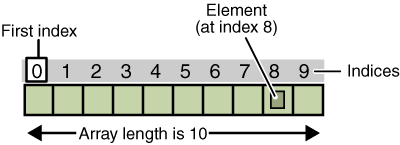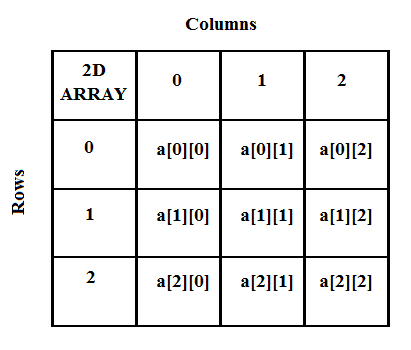# Arrays in C Programming

An array is a collection of data of the same type. One could imagine them to be a collection of variables of a particular type.

User can declare arrays of a given size(say$n$) and name. An array can thus be thought of containing$n$ values or values of$n$ variables.

Each entry or element of an array can be accessed by it’s index.
All arrays consist of contiguous memory locations. The lowest address corresponds to the first element and the highest address to the last element.### Declaring an array:

Arrays can be declared by specifying the data-type, name and the size.
Ex:

```//An integer array of size 10 and name 'digits'
int digits;
//An array of type double, size 5 and name 'numbers'
double numbers;
```

All the above are examples of one-dimensional arrays.

### Initializing the arrays:

There are two ways, to initialize an array.
Do it at the time of declaration:

```double balance = {1000.0, 2.0, 3.4, 7.0, 50.0};
```

One can even omit the size if the array is being initialized when it is being declared.
Ex:

```double balance[] = {1000.0, 2.0, 3.4, 7.0, 50.0};
```

This will create an array just big enough to hold the initialization.

The other way, is to first declare an array is shown before, and then initialize each index separately.
Ex:

```double marks;
marks=96.5;
marks=93.5;
marks=91;
marks=93;
```

Note: In the above code, you can notice, that the array indices start from 0. So the index of the nth element is n-1.
For an array of size 10, the index of the last entry would be 9.
Just keep this info in mind for the future.

### Accessing Array Elements:

One can access an element of the array by specifying the array name and the index of the element.
Ex:

```double temp = balance;
```

The above statement will take the 10th element from the array and assign the value to temp variable.

### Multi-Dimensional Arrays

C programming language allows multidimensional arrays. The general form of a multidimensional array declaration-

```type arrayName[size1][size2][size3]....[sizeN];
```

Thus, a multi-dimensional array is basically an array of arrays.

### Two-Dimensional(2D) Arrays

2D arrays are important from mathematical point of view as they can be interpreted as matrices.

#### Declaring 2D arrays

One can declare 2D arrays by specifying the type, name and the size of the 2 dimensions.
Ex:

```int matrix;
```

Basically, this means that we declare a 2D array of type int, and name ‘matrix’, and size 2×3.
This can be thought of as being a table/matrix which will have 2 rows and 3 columns.
One could consider it to have 2 arrays each of size 4.#### Initializing 2D arrays

Like, before there are again two ways to initialize a 2D array.
You can do it all at once, by enclosing the elements of a row within curly braces:
Ex:

```int a = {
{0, 1, 2, 3} ,   /*  initializers for row indexed by 0 */
{4, 5, 6, 7} ,   /*  initializers for row indexed by 1 */
{8, 9, 10, 11}   /*  initializers for row indexed by 2 */
};
```

or you can initialize each element separately, using their indices.

```a=4;
```

Note:
The nested braces, which indicate the intended row, are optional. They are just used for better representation. The following initialization is equivalent to the previous example −

```int a = {0,1,2,3,4,5,6,7,8,9,10,11};
```

#### Accessing elements of 2D arrays

Like 1D arrays, the elements can be accessed as shown:

```double val=matrix;
```

This will assign the value of the element in the first row, and second column to the variable val.

Well, I hope that clears up the concept of arrays in C programming.
We will be using this a lot in the future posts, as we will write a lot of programs on matrix operations.

Hope you found it useful.
If you have any questions or doubts, drop them in the comments section down below.[wpedon id="7041" align="center"]